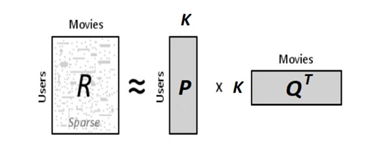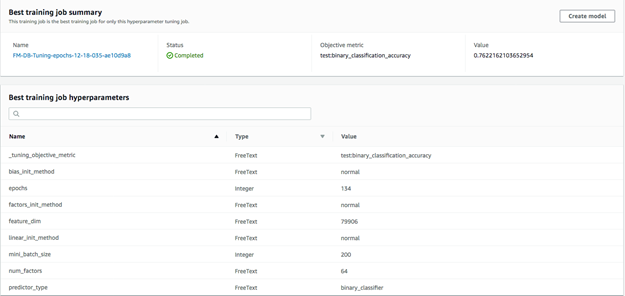# 使用AWS Sagemaker训练因子分解机模型并应用于推荐系统

### 使用AWS Sagemaker系列文章：

————## 数据准备阶段

In :

``````df_all = pd.read_csv('all_movie_rates_noblank.csv')
print(df_all.shape)
print(df_all['UserId'].nunique())
print(df_all['MovieID'].nunique())``````

(3963891, 3)

490790

33345

In :

``````df=pd.read_csv('all_movie_rates_100k.csv')
print(df.shape)
print(df['UserId'].nunique())
print(df['MovieID'].nunique())

df_train, df_test = train_test_split(df, test_size=0.2, random_state=42)
print(df_train.shape, df_test.shape)

(107029, 3)

53902

26004

(85623, 3) (21406, 3)

MovieID  Rate           UserId

63287  1296827    10      BloodzBoi

76527  6874441     8       49886917

71411  3152563     8     feathercat

64499  2004250     6  jingtianwst83

42130  3230459     6          funni

88502  3313801     6     chrisocean

79349  3072140     0       lala1123

21431  2053746     6      145992805

39240  1464338     8   HeroineDaode

MovieID  Rate        UserId

44496  26304167     8   152833029

11855   1299900     8      leonah

13955  26085750     2    49298107

27112   4202982     8  Kylin-2015

28103   4739952    10      likong

53598   3443393     4     3832465

65338   2052363     6     1926472

1116    6129707     8  vero_nicat

38854  26841337     2   158039357

78721   1307026     8    TowaErio

### 为用户和电影分别建立字典（Python Dictionary）

In :

``````filename = 'all_movie_rates_100k.csv'
user_number = 53902
movie_number = 26004

def createIDtoIndexDict(filename, user_number, movie_number):
u_Dict = {}
m_Dict = {}
i = 0
m = 0
with open(filename, 'r') as f:
for MovieID, Rate, UserId in sample:
if UserId == 'UserId':
continue
else:
if UserId not in u_Dict.keys():
u_Dict[UserId] = i
i = i+1
if MovieID not in m_Dict.keys():
m_Dict[MovieID] = m
m = m+1
return u_Dict, m_Dict
u_Dict, m_Dict = createIDtoIndexDict(filename, user_number, movie_number)
``````

### 建立稀疏矩阵（Sparse Matrix）和标签向量（Label Vector）

``````df_train.to_csv('100k_train.csv', index=False, encoding="utf_8_sig")
df_test.to_csv('100k_test.csv', index=False, encoding="utf_8_sig")

columns = user_number+movie_number
X = scipy.sparse.lil_matrix((lines, columns)).astype('float32')
Y = []
line = 0
with open (filename, 'r') as f:
for MovieID, Rate, UserId in sample:
if UserId == 'UserId':
continue
else:
X[line, u_Dict[UserId]] = 1
X[line, user_number+m_Dict[MovieID]] = 1
if Rate == 'Rate' or int(Rate) < 6:
Y.append(0)
else:
Y.append(1)
line=line+1

Y=np.array(Y).astype('float32')
print(X.shape)
print(Y.shape)
return X, Y
``````

In :

``````X_train, Y_train = loadDataset('100k_train.csv', df_train.shape, columns)
X_test, Y_test = loadDataset('100k_test.csv', df_test.shape, columns)
print(X_train.shape, X_test.shape)
print(Y_train.shape, Y_test.shape)
``````

(85623, 79906)

(85623,)

(21406, 79906)

(21406,)

(85623, 79906) (21406, 79906)

(85623,) (21406,)

### 转换稀疏矩阵为protobuf格式，并保存到S3

In :

``````bucket = 'movie-recommendation-demo-raw-data'
prefix = 'sagemaker/movie-recommendation'
train_key      = 'train.protobuf'
train_prefix   = '{}/{}'.format(prefix, 'train')
test_key       = 'test.protobuf'
test_prefix    = '{}/{}'.format(prefix, 'test')
output_prefix  = 's3://{}/{}/output'.format(bucket, prefix)

def writeDatasetToProtobuf(X, Y, bucket, prefix, key):
import io,boto3
import sagemaker.amazon.common as smac
buf = io.BytesIO()
smac.write_spmatrix_to_sparse_tensor(buf, X, Y)
buf.seek(0)
print(buf)
obj = '{}/{}'.format(prefix, key)
print('Wrote dataset: {}/{}'.format(bucket,obj))
return 's3://{}/{}'.format(bucket,obj)

train_data = writeDatasetToProtobuf(X_train, Y_train, bucket, train_prefix, train_key)
test_data = writeDatasetToProtobuf(X_test, Y_test, bucket, test_prefix, test_key)

print(train_data)
print(test_data)
print('Output: {}'.format(output_prefix))
``````

<_io.BytesIO object at 0x7f99a8b8e1a8>

Wrote dataset: movie-recommendation-demo-raw-data/sagemaker/movie-recommendation/train/train.protobuf

<_io.BytesIO object at 0x7f99a8b8e1a8>

Wrote dataset: movie-recommendation-demo-raw-data/sagemaker/movie-recommendation/test/test.protobuf

s3://movie-recommendation-demo-raw-data/sagemaker/movie-recommendation/train/train.protobuf

s3://movie-recommendation-demo-raw-data/sagemaker/movie-recommendation/test/test.protobuf

Output: s3://movie-recommendation-demo-raw-data/sagemaker/movie-recommendation/output

## FM模型训练

FM是SageMaker自带的算法之一，因此通过SageMaker训练模型非常容易。首先我们需要引入SageMaker的SDK，并建立SageMaker的session、定义位于该Region的因子分解机算法Container以及获取SageMaker的运行角色。

In :

``````from sagemaker import get_execution_role
from sagemaker.amazon.amazon_estimator import get_image_uri
import sagemaker
sess = sagemaker.Session()

role =  get_execution_role()
container = get_image_uri(boto3.Session().region_name, 'factorization-machines')
``````

In :

``````fm = sagemaker.estimator.Estimator(container,
role,
train_instance_count=1,
train_instance_type='ml.c4.xlarge',
output_path=output_prefix,
sagemaker_session=sess)
fm.set_hyperparameters(feature_dim=79906,
predictor_type='binary_classifier',
mini_batch_size=1000,
num_factors=64,
epochs=50)

fm.fit({'train': train_data, 'test':test_data})
``````

INFO:sagemaker:Creating training-job with name: factorization-machines-2018-12-18-03-18-50-388

2018-12-18 03:18:50 Starting – Starting the training job…

2018-12-18 03:18:56 Starting – Launching requested ML instances……

2018-12-18 03:19:58 Starting – Preparing the instances for training…

Docker entrypoint called with argument(s): train

[12/18/2018 03:22:09 INFO 139830159329088] #quality_metric: host=algo-1, test binary_classification_accuracy <score>=0.736288890965

[12/18/2018 03:22:09 INFO 139830159329088] #quality_metric: host=algo-1, test binary_classification_cross_entropy <loss>=0.548373071499

[12/18/2018 03:22:09 INFO 139830159329088] #quality_metric: host=algo-1, test binary_f_1.000 <score>=0.84806072188

[2018-12-18 03:22:09.767] [tensorio] [info] data_pipeline_stats={“name”: “/opt/ml/input/data/test”, “epoch”: 1, “duration”: 366, “num_examples”: 22}

[2018-12-18 03:22:09.767] [tensorio] [info] data_pipeline_stats={“name”: “/opt/ml/input/data/test”, “duration”: 40228, “num_epochs”: 2, “num_examples”: 23}

#metrics {“Metrics”: {“totaltime”: {“count”: 1, “max”: 40280.484199523926, “sum”: 40280.484199523926, “min”: 40280.484199523926}, “setuptime”: {“count”: 1, “max”: 39.59202766418457, “sum”: 39.59202766418457, “min”: 39.59202766418457}}, “EndTime”: 1545103329.767301, “Dimensions”: {“Host”: “algo-1”, “Operation”: “training”, “Algorithm”: “factorization-machines”}, “StartTime”: 1545103329.299489}

[2018-12-18 03:22:09.784] [tensorio] [info] data_pipeline_stats={“name”: “/opt/ml/input/data/train”, “epoch”: 50, “duration”: 1287, “num_examples”: 86}

[2018-12-18 03:22:09.784] [tensorio] [info] data_pipeline_stats={“name”: “/opt/ml/input/data/train”, “duration”: 39923, “num_epochs”: 51, “num_examples”: 4301}

Billable seconds: 92

• 模型训练计费时间92秒，所以并不会花很多钱；
• 模型的二分准确度为73.62%In :

``````fm.set_hyperparameters(feature_dim=79906,
predictor_type='binary_classifier',
mini_batch_size=200,
num_factors=64,
epochs=134)
``````

In :

``````fm_predictor = fm.deploy(initial_instance_count=1,
instance_type='ml.t2.medium')
``````

INFO:sagemaker:Creating model with name: factorization-machines-2018-12-18-05-56-31-040

INFO:sagemaker:Creating endpoint with name factorization-machines-2018-12-18-05-47-26-108

—————————————————————–!

————

## 本篇作者### 崔辰

AWS大中华区创新中心技术业务拓展经理。加入AWS之前，崔辰在中国惠普、IBM、微软以及海航科技等公司担任过售前技术顾问、市场经理和战略合作经理等职务。在10多年的科技领域工作经历中，崔辰服务过众多企业级客户。

TAGS: